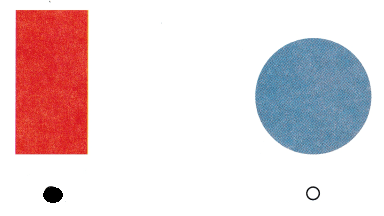# Texas Go Math Kindergarten Lesson 17.1 Answer Key Identify Circles

Refer to our Texas Go Math Kindergarten Answer Key Pdf to score good marks in the exams. Test yourself by practicing the problems from Texas Go Math Kindergarten Lesson 17.1 Answer Key Identify Circles.

## Texas Go Math Kindergarten Lesson 17.1 Answer Key Identify Circles

ExploreExplanation:
A circle is a shape consisting of all points in a plane that are at a given distance from a given point, the center.

Directions
Use your finger to trace around the circle. Talk about the curve. Use a pencil or crayon to trace around the circle.

Share and Show

Question 1.Explanation:
A circle has a curved surface
and a circle doesn’t have sides.

Directions
1. Use two-dimensional shapes. Sort the shapes by circles and not circles. Trace and color the shapes on the sorting mat.

Question 2.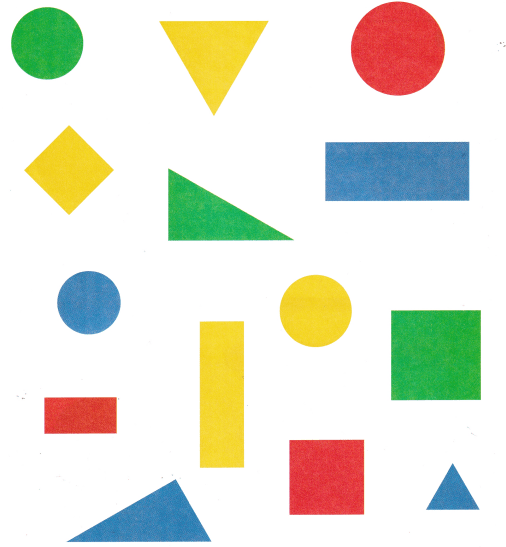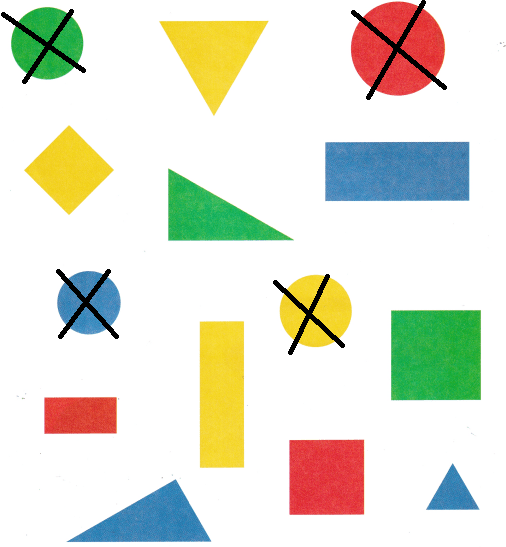Explanation:
A circle consists of a closed curved line around a central point.
so, crossed all the circles

Directions
2. Mark an X on all of the circles.

Home Activity

• Have your child show you an object that is shaped like a circle.

Problem Solving

Question 3.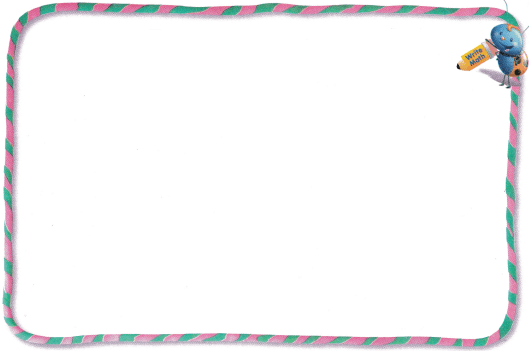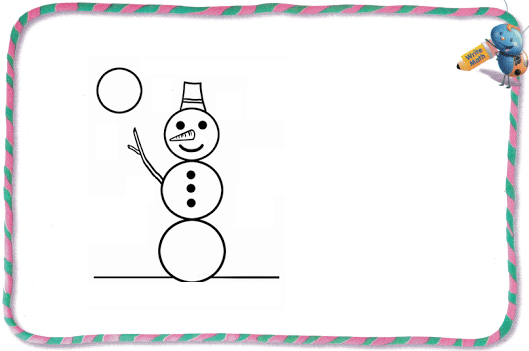Explanation:
An ice man is prepared with the help of the circles
a circle has a curved surface.

Question 4.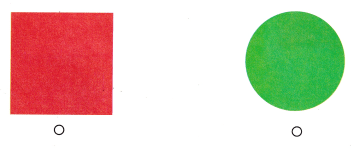Explanation:
The first figure is a rectangle has flat surface.
second figure is a circle and it has curved surface.
so, marked the circle.

Directions
3. Look at your shapes. Draw the shape that has a circle. Tell a friend the name of the shape. 4. Choose the correct answer. Which shape has a curved edge?

### Texas Go Math Kindergarten Lesson 17.1 Homework and Practice

Question 1.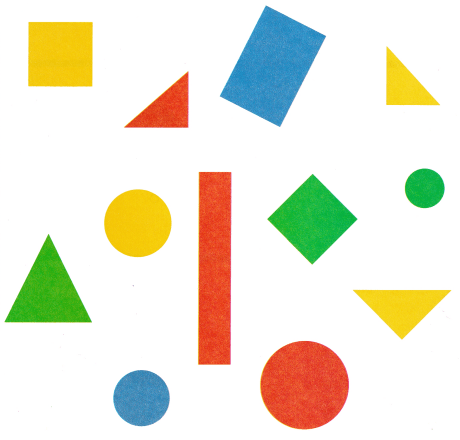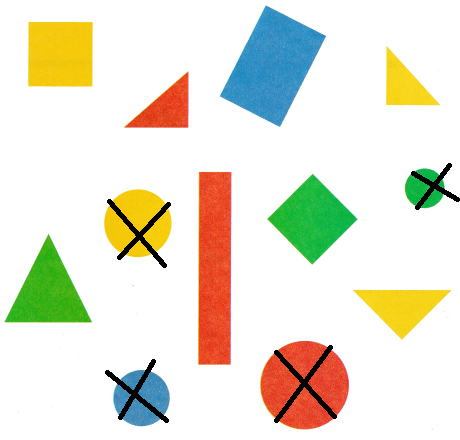Explanation:
A circle consists of a closed curved line around a central point.
so, crossed all the circles

Directions
1. Mark on X on all of the circles.

Texas Test Prep

Lesson Check

Question 2.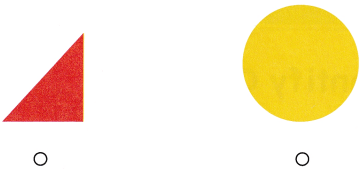Explanation:
The first figure is a triangle has flat surface.
second figure is a circle and it has curved surface.
so, marked the circle.

Question 3.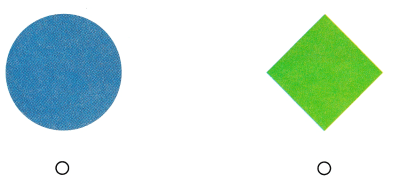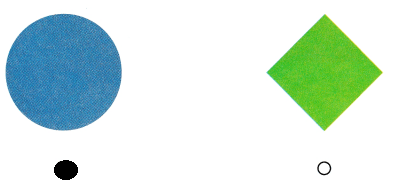Explanation:
The second figure is a rhombus has flat surface.
first figure is a circle and it has curved surface.
so, marked the circle.

Question 4.